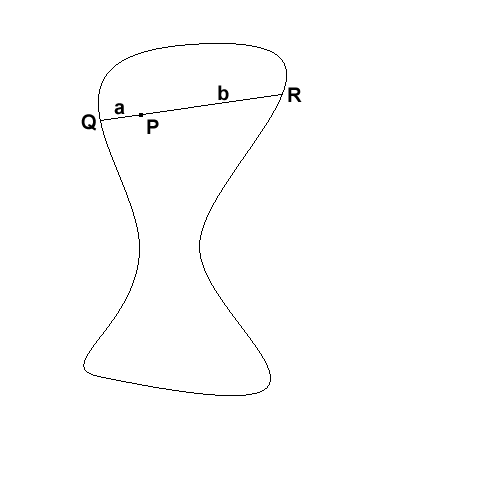#### You may also like### Areas and Ratios

Do you have enough information to work out the area of the shaded quadrilateral?### Shape and Territory

If for any triangle ABC tan(A - B) + tan(B - C) + tan(C - A) = 0 what can you say about the triangle?### Napoleon's Hat

Three equilateral triangles ABC, AYX and XZB are drawn with the point X a moveable point on AB. The points P, Q and R are the centres of the three triangles. What can you say about triangle PQR?

# Middle Man

##### Age 16 to 18Challenge Level

Amir sent us this solution:Draw any chord passing through $P$, and call its endpoints $Q$ and $R$. Let $a$ be the length of the line segment $PQ$, and $b$ the length of $PR$.

If $a=b$, then clearly $P$ is the midpoint of a chord, so we're done. So suppose that $a\neq b$. We may as well assume that $a< b$ (otherwise just switch round $Q$ and $R$). Imagine that the shape is made out of a metal frame, and that the chord $QR$ is made from elastic, just looped round the frame at $Q$ and at $R$, but fixed at $P$ (so that it can rotate). Rotate the chord around $P$, and the elastic will stretch so that the line is always a chord of the shape. When it's gone 180$^{\circ}$ round, $QP$ will have length $b$, and $PR$ will have length $a$, in other words, the segments will have switched. So now $|QP|> |PR|$, when they started the other way round. But as we turn the chord, the lengths of the segments change continuously, so to switch from $QP$ being shorter to $QP$ being longer, we must have had $|QP|=|QR|$ at some point. But then $P$ will be the midpoint of this chord.